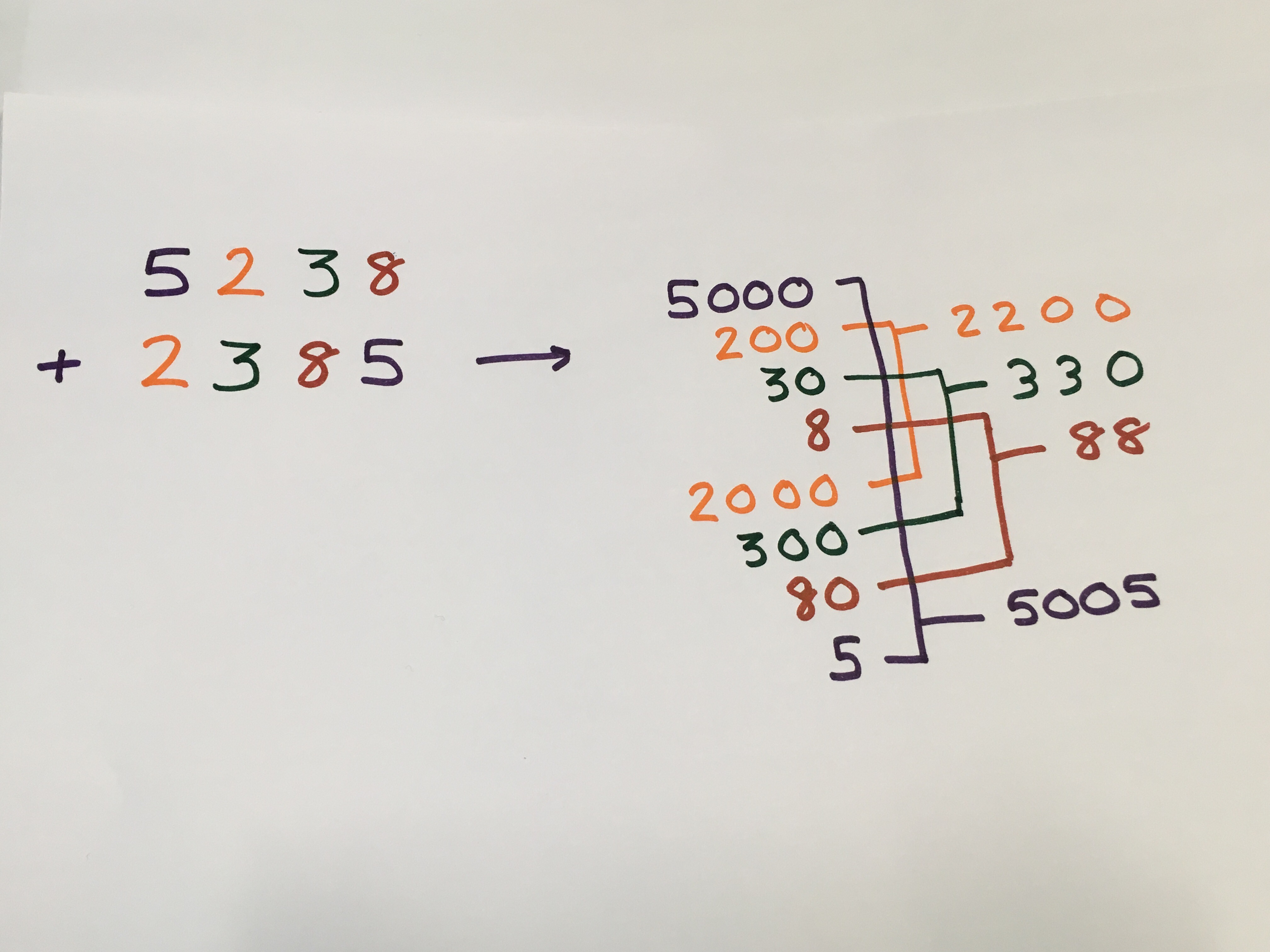#### You may also likeMake a set of numbers that use all the digits from 1 to 9, once and once only. Add them up. The result is divisible by 9. Add each of the digits in the new number. What is their sum? Now try some other possibilities for yourself!### Counting Factors

Is there an efficient way to work out how many factors a large number has?### Repeaters

Choose any 3 digits and make a 6 digit number by repeating the 3 digits in the same order (e.g. 594594). Explain why whatever digits you choose the number will always be divisible by 7, 11 and 13.

# Legs Eleven

### Why do this problem?

The unexpected result is a great hook that can be used to engage curious students.

Challenging your students to justify and explain their findings will encourage them to develop their understanding of place value and their reasoning skills at the same time.

The problem may be suitable for students to work on in small groups.

### Possible approach

Share the animation with students and ask them to describe what they notice.

"I think the answer is divisible by 11. How can we check?"

Your students may wish to use a division algorithm here but you may like to draw their attention to the fact that we only wish to confirm that there is no remainder, not find the answer to the division. One way to do this is to subtract known multiples of eleven for example:

7623 - 6600 = 1023
1023 - 22 = 1001

If we can confirm that 1001 is divisible by 11 then we know 7623 is divisible by 11.

"Let's try some more examples...
Choose your own 4 digit number, move the first digit to the back of the queue and add the result to your original number. Check whether your answer is divisible by 11."

This is a good moment for self checking as if anyone thinks they have a counter example you could do it on the board altogether and this can help to sort out any mistakes in their addition strategy or misconceptions about divisibility.

"Will it always work?"

Give students some time to think about this. It might be a good time to work in groups. You could share the prompt below.

"If I started with 5 thousands, 2 hundreds, 3 tens and 8 units.
We could write it as
$5\times1000 + 2\times100 + 3\times10 + 8\times1$

After I moved the digits along, my new number would have 2 thousands, 3 hundreds, 8 tens and 5 units, and could be written as
$2\times1000 + 3\times100 + 8\times10 + 5\times1$

How many 5s, 2s, 3s and 8s would there be when we add the two numbers?"Your students may come up with a variety of explanations.

One way might be to decompose each number into its place value parts and recombine the parts from the two numbers.

If we can convince ourselves that these are all multiples of 11 we know the total must be a multiple of 11.

### Key questions

How do we know a number is divisible by 11?

Will this process always generate a multiple of 11?
How can we prove that it will always work?

### Possible support

You might like to look at Always a Multiple? first. There are similarities between these problems as they both focus on the importance of place value and they both have links to the 11 times table.